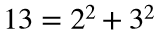# Problem 49738. Determine whether a prime is Pythagorean

Pythagorean primes get their name from the property that they can be written as the sum of two squares. For example,. Cody Problem 45964 asked you to find the nth Pythagorean prime and two numbers whose squares will produce the prime. This problem merely asks you to determine whether a prime can be written as the sum of two squares.
Write a function to determine whether a prime is Pythagorean. All input numbers in the tests are prime. Because some are large, they are given as strings.

### Solution Stats

31.25% Correct | 68.75% Incorrect
Last Solution submitted on Aug 19, 2023

### Community Treasure Hunt

Find the treasures in MATLAB Central and discover how the community can help you!

Start Hunting!